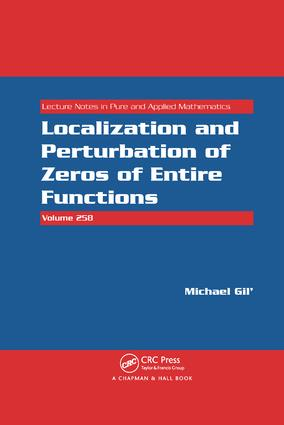# Localization and Perturbation of Zeros of Entire Functions

## 1st Edition

Chapman and Hall/CRC

312 pages

##### Purchasing Options:\$ = USD
Paperback: 9781138116788
pub: 2017-06-07
\$82.95
x
Hardback: 9781439800324
pub: 2009-12-04
\$255.00
x
eBook (VitalSource) : 9780429131882
pub: 2009-12-04
from \$39.98

FREE Standard Shipping!

### Description

One of the most important problems in the theory of entire functions is the distribution of the zeros of entire functions. Localization and Perturbation of Zeros of Entire Functions is the first book to provide a systematic exposition of the bounds for the zeros of entire functions and variations of zeros under perturbations. It also offers a new approach to the investigation of entire functions based on recent estimates for the resolvents of compact operators.

After presenting results about finite matrices and the spectral theory of compact operators in a Hilbert space, the book covers the basic concepts and classical theorems of the theory of entire functions. It discusses various inequalities for the zeros of polynomials, inequalities for the counting function of the zeros, and the variations of the zeros of finite-order entire functions under perturbations. The text then develops the perturbation results in the case of entire functions whose order is less than two, presents results on exponential-type entire functions, and obtains explicit bounds for the zeros of quasipolynomials. The author also offers additional results on the zeros of entire functions and explores polynomials with matrix coefficients, before concluding with entire matrix-valued functions.

This work is one of the first to systematically take the operator approach to the theory of analytic functions.

Finite Matrices

Inequalities for eigenvalues and singular numbers

Inequalities for convex functions

Traces of powers of matrices

A relation between determinants and resolvents

Estimates for norms of resolvents in terms of the distance to spectrum

Bounds for roots of some scalar equations

Perturbations of matrices

Preservation of multiplicities of eigenvalues

An identity for imaginary parts of eigenvalues

Additional estimates for resolvents

Gerschgorin’s circle theorem

Cassini ovals and related results

The Brauer and Perron theorems

Eigenvalues of Compact Operators

Banach and Hilbert spaces

Linear operators

Classification of spectra

Compact operators in a Hilbert space

Compact matrices

Resolvents of Hilbert–Schmidt operators

Operators with Hilbert–Schmidt powers

Resolvents of Schatten–von Neumann operators

Auxiliary results

Equalities for eigenvalues

Proofs of Theorems 2.6.1 and 2.8.1

Spectral variations

Preservation of multiplicities of eigenvalues

Entire Banach-valued functions and regularized determinants

Some Basic Results of the Theory of Analytic Functions

The Rouché and Hurwitz theorems

The Caratheodory inequalities

Jensen’s theorem

Lower bounds for moduli of holomorphic functions

Order and type of an entire function

Taylor coefficients of an entire function

The theorem of Weierstrass

Density of zeros

An estimate for canonical products in terms of counting functions

The convergence exponent of zeros

The Borel transform

Polynomials

Some classical theorems

Equalities for real and imaginary parts of zeros

Partial sums of zeros and the counting function

Sums of powers of zeros

The Ostrowski-type inequalities

Proof of Theorem 4.5.1

Higher powers of real parts of zeros

The Gerschgorin type sets for polynomials

Perturbations of polynomials

Proof of Theorem 4.9.1

Preservation of multiplicities

Distances between zeros and critical points

Partial sums of imaginary parts of zeros

Functions holomorphic on a circle

Bounds for Zeros of Entire Functions

Partial sums of zeros

Proof of Theorem 5.1.1

Functions represented in the root-factorial form

Functions represented in the Mittag–Leffler form

An additional bound for the series of absolute values of zeros

Proofs of Theorems 5.5.1 and 5.5.3

Partial sums of imaginary parts of zeros

Representation of ezr in the root-factorial form

The generalized Cauchy theorem for entire functions

The Gerschgorin-type domains for entire functions

The series of powers of zeros and traces of matrices

Zero-free sets

Taylor coefficients of some infinite-order entire functions

Perturbations of Finite-Order Entire Functions

Variations of zeros

Proof of Theorem 6.1.2

Approximations by partial sums

Preservation of multiplicities

Distances between roots and critical points

Tails of Taylor series

Functions of Order Less than Two

Relations between real and imaginary parts of zeros

Proof of Theorem 7.1.1

Perturbations of functions of order less than two

Proof of Theorem 7.3.1

Approximations by polynomials

Preservation of multiplicities of in the case p(f) < 2

Exponential-Type Functions

Application of the Borel transform

The counting function

The case a(f) < ∞

Variations of roots

Functions close to cos z and ez

Estimates for functions on the positive half-line

Difference equations

Quasipolynomials

Sums of absolute values of zeros

Variations of roots

Trigonometric polynomials

Estimates for quasipolynomials on the positive half-line

Differential equations

Positive Green functions of functional differential equations

Stability conditions and lower bounds for quasipolynomials

Transforms of Finite-Order Entire Functions and Canonical Products

Comparison functions

Transforms of entire functions

Relations between canonical products and Sp

Lower bounds for canonical products in terms of Sp

Proof of Theorem 10.4.1

Canonical products and determinants

Perturbations of canonical products

Polynomials with Matrix Coefficients

Partial sums of moduli of characteristic values

An identity for sums of characteristic values

Imaginary parts of characteristic values of polynomial pencils

Perturbations of polynomial pencils

Multiplicative representations of rational pencils

The Cauchy type theorem for polynomial pencils

The Gerschgorin type sets for polynomial pencils

Estimates for rational matrix functions

Coupled systems of polynomial equations

Vector difference equations

Entire Matrix-Valued Functions

Preliminaries

Partial sums of moduli of characteristic values

Proof of Theorem 12.2.1

Imaginary parts of characteristic values of entire pencils

Variations of characteristic values of entire pencils

Proof of Theorem 12.5.1

An identity for powers of characteristic values

Multiplicative representations of meromorphic matrix functions

Estimates for meromorphic matrix functions

Zero free domains

Matrix-valued functions of a matrix argument

Green’s functions of differential equations

Bibliography

Index

### About the Author

Michael Gil’ is a professor in the Department of Mathematics at Ben Gurion University of the Negev in Israel.

### Subject Categories

##### BISAC Subject Codes/Headings:
MAT037000
MATHEMATICS / Functional Analysis